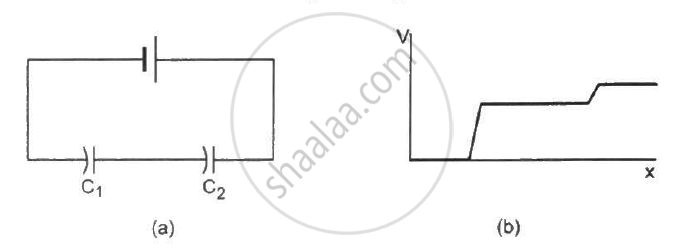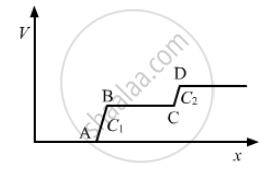Department of Pre-University Education, Karnataka course PUC Karnataka Science Class 12
Share

# P Figure (31-q3) Shows Two Capacitors Connected in Series and Joined to a Battery. the Graph Shows the Variation in Potential as One Moves from Left to Right on the Branch Containing the Capacitors. - Physics

ConceptCombination of Capacitors

#### Question

The following figure shows two capacitors connected in series and joined to a battery. The graph shows the variation in potential as one moves from left to right on the branch containing the capacitors.• C1 > C2

• C1 = C2

• C1 < C2

• The information is not sufficient to decide the relation between C1 and C2.

#### Solution

C1 < C​2Region AB shows the potential difference across capacitor C​1 and region CD shows the potential difference across capacitor C2. Now, we can see from the graph that region AB is greater than region CD. Therefore, the potential difference across capacitor C1 is greater than that across capacitor C2.

∵ Capacitance, C = Q/V

∴ C​​1 < C2         (Q remains the same in series connection.)

Is there an error in this question or solution?

#### Video TutorialsVIEW ALL 

Solution P Figure (31-q3) Shows Two Capacitors Connected in Series and Joined to a Battery. the Graph Shows the Variation in Potential as One Moves from Left to Right on the Branch Containing the Capacitors. Concept: Combination of Capacitors.
S